Next: Wisconsin Epidemiological Study of Up: Examples Previous: Chickenpox Epidemic

## Lake Acidity Study

This data set was derived by Douglas and Delampady (1990) from the Eastern Lakes Survey of 1984. It contains measurements of 1789 lakes in three Eastern US regions: Northeast, Upper Midwest and Southeast. Of interest is the dependence of the water pH level (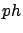) on the calcium concentration inmilligrams per liter (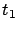) and the geographical location (where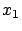=latitude and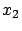=longitude). Gu and Wahba (1993a) analyzed this data set using SS ANOVA models. As in Gu and Wahba (1993a), we use a subset of 112 lakes in the southern Blue Ridge mountains area.

We use this data set to illustrate how to fit a cubic spline, a cubic spline with correlated random errors, a SLM and an SS ANOVA model.

First, we fit a cubic spline tousing one variable calcium ()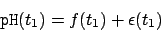where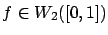.

> acid.fit1 <- ssr(ph~t1, rk=cubic(t1), data=acid, scale=T)
> summary(acid.fit1)
...
GCV estimate(s) of smoothing parameter(s) : 3.84e-06
Equivalent Degrees of Freedom (DF):  8.21
Estimate of sigma:  0.281
> anova(acid.fit1)

Testing H_0: f in the NULL space

test.value simu.size simu.p-value
LMP 0.003634651       100         0.04
GCV 0.008239078       100         0.02


Both p-values from the LMP and GCV tests are small, indicating that a simple linear model is not sufficient to describe the relationship. This can be confirmed by looking at the fitted function and its projections onto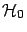and. A linear model is equivalent to the projection ontobeing zero. The following statements compute posterior means and standard deviations for the projections ontowith terms=c(1,1,0), the projections ontowith terms=c(0,0,1), and the overall fit with terms=c(1,1,1).


> grid <- data.frame(t1=seq(min(acid$t1), max(acid$t1), len=100))
> tm <- matrix(c(1,1,0,0,0,1,1,1,1), ncol=3, byrow=T)
> p.acid.fit1 <- predict(acid.fit1, grid, terms=tm)


Figureshows the fitted function and its projections. The nonlinear part is small, but different from zero.pH observations close in geographic locations are often correlated. Suppose that we want to use spherical spatial correlation structure with nugget effect for the variable location. That is, regard random errors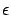as a function of, and assume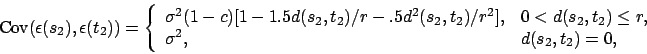where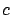is the nugget effect,is the Euclidean distance between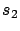and, andis the range parameter.

> acid$s1 <- (acid$t1-min(acid$t1))/diff(range(acid$t1))
> acid.fit2 <- ssr(ph~s1, rk=cubic(s1), data=acid,
corr=corSpher(form=~x1+x2,nugget=T), spar="m")
> acid.fit2
...
Coefficients (d):
(Intercept)          s1
6.221757    1.264431

GML estimate(s) of smoothing parameter(s) : 4.645508
Equivalent Degrees of Freedom (DF):  2.000284
Estimate of sigma:  0.2994075
Correlation structure of class corSpher representing
range     nugget
0.03646616 0.68530949


We plot in Figurefitted curves from acid.fit1, acid.fit2 and acid.fit5. Notice the effect of the correlation on the smoothing parameter and the fit. Equivalent degrees of freedom fordecreased from 8.21 to 2.00. The new fit is linear. The smaller smoothing parameter in the first fit might be caused by the spatial correlation.We can also model the effect of location directly as a covariate. We consider two approaches to model location effect: use random effects with exponential spatial correlation structure (similar to Kriging), and use thin plate splines.

For the first approach, we consider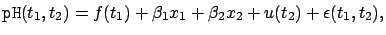(76)

where,is a spatial process and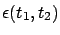are random errors independent of the spatial process. Model () separates the contribution of the spatial correlation to random errors in acid.fit2 from other sources, and regard the spatial process as part of the signal. To show different options, we now assume an exponential correlation structure. Specifically, we assumein () is a mean zero process withwhere,andare the same as those defined in the spherical correlation structure. Note that we include the linear effects of location in the fixed effects. This will allow us to compare with the fit of a thin plate spline model later.

Denote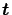as the vector of design points for. Letbe the vector of the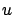process evaluated at design points.are random effects and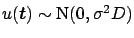, where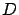depends parametersand. Again, the SLM model () cannot be fitted directly using slm sincedepends on the range parameternonlinearly. We fit model () in two steps. We first regardas part of random error, estimate the range parameter, and calculate the estimated covariance matrix without nugget effect:

> temp <- ssr(ph~t1+x1+x2, rk=tp(t1), data=acid,
corr=corExp(form=~x1+x2, nugget=T), spar="m")
> tau <- coef(temp$cor.est, F) > D <- corMatrix(initialize(corExp(tau,form=~x1+x2), data=acid))  Consider the estimatedas the true covariance matrix. Then we can calculate the Cholesky decomposition ofas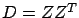, and transform the random effects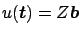, where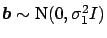. Now we are ready to fit the transformed SLM: > Z <- chol.new(D) > acid.fit3 <- slm(ph~t1+x1+x2, rk=tp(t1), data=acid, random=list(pdIdent(~Z-1))) > summary(acid.fit3) ... Coefficients (d): (Intercept) t1 x1 x2 6.5483884 0.6795706 -8.3694493 2.1135023 GML estimate(s) of smoothing parameter(s) : 0.0005272899 Equivalent Degrees of Freedom (DF): 4.000179 Estimate of sigma: 0.002725062  We then calculate the estimated effect of calcium: grid1 <- data.frame(t1=seq(min(acid$t1), max(acid$t1), len=100), x1=min(acid$x1), x2=min(acid$x2)) p.acid.fit3.t1 <- intervals(acid.fit3, grid1, terms=c(0,1,0,0,1))  Suppose that we want to calculate the location effect on grid points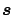. Let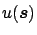be the vector of theprocess evaluated at elements in. Let. Then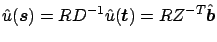. grid2 <- expand.grid( x1=seq(min(acid$x1)-.001,max(acid$x1)+.001, len=20), x2=seq(min(acid$x2)-.001,max(acid$x2)+.001, len=20)) newdata <- data.frame(y1=c(acid$x1,grid2$x1), y2=c(acid$x2,grid2$x2)) RD <- corMatrix(initialize(corExp(tau, form=~y1+y2), data=newdata)) R <- RD[(length(acid$x1)+1):length(newdata$y1),1:length(acid$x1)]
u.new <- R%*%t(solve(Z))%*%as.vector(acid.fit3$lme.obj$coef$random[]) p.acid.fit3.t2 <- acid.fit3$lme.obj$coef$fixed*grid2$x1+ acid.fit3$lme.obj$coef$fixed*grid2$x2+u.new  Figureplots the estimated main effects ofandon the left panel. As the second approach, we consider the same thin plate spline model as in Gu and Wahba (1993a). Specifically, we use a TPS with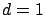and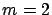to model the effect of calcium, and a TPS with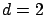andto model the effect of location. Then we have the following SS ANOVA model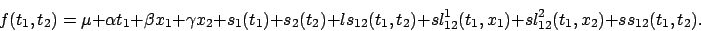Components on the right hand side are constant, linear main effect of, linear main effect of, linear main effect of, smooth main effect of, smooth main effect of, linear-smooth interaction betweenand, smooth-linear interaction betweenand, smooth-linear interaction betweenand, and smooth-smooth interaction betweenand. > acid.fit4 <- ssr(ph~t1+x1+x2, rk=list(tp(t1), tp(list(x1,x2)), rk.prod(kron(t1),tp(list(x1,x2))), rk.prod(kron(x1),tp(t1)), rk.prod(kron(x2),tp(t1)), rk.prod(tp(t1),tp(list(x1,x2)))), data=acid, spar="m") > summary(acid.fit4) ... Coefficients (d): (Intercept) t1 x1 x2 6.555506 0.6235892 -8.783944 1.974596 GML estimate(s) of smoothing parameter(s) : 1.816737e+04 3.600865e-03 2.710276e+01 2.759507e+00 1.992876e+01 1.440841e-01 Equivalent Degrees of Freedom (DF): 10.7211 Estimate of sigma: 0.2560693  Since the smoothing parameters corresponding to the interaction terms are large, it is easy to check that these interaction terms are small. Thus we reduce to the following additive model with main effects only> acid.fit5 <- update(acid.fit3, rk=list(tp(t1), tp(list(x1,x2)))) > summary(acid.fit5) ... ... Coefficients (d): (Intercept) t1 x1 x2 6.555502 0.6235885 -8.783569 1.974339 GML estimate(s) of smoothing parameter(s) : 6.045750e+03 3.600423e-03 Equivalent Degrees of Freedom (DF): 10.72108 Estimate of sigma: 0.2560684 > grid3 <- data.frame(t1=seq(min(acid$t1), max(acid$t1), len=100), x1=min(acid$x1), x2=min(acid$x2)) > p.acid.fit5.t1 <- predict(acid.fit5, grid3, terms=c(0,1,0,0,1,0)) > grid4 <- expand.grid(t1=min(acid$t1),
x1=seq(min(acid$x1), max(acid$x1), len=20),
x2=seq(min(acid$x2), max(acid$x2), len=20))
> p.acid.fit5.t2 <- predict(acid.fit5, grid4, terms=c(0,0,1,1,0,1))


Figureplots the estimated main effects ofandon the right panel. It is seen that the estimates of the calcium main effects are almost identical. The estimates of the location main effects have a similar pattern. The estimate from acid.fit5 is smoother.

> grid3 <- data.frame(t1=seq(min(acid$t1), max(acid$t1), len=100),
x1=min(acid$x1), x2=min(acid$x2))
> p.acid.fit5.t1 <- predict(acid.fit5, grid3, terms=c(0,1,0,0,1,0))
> grid4 <- expand.grid(t1=min(acid$t1), x1=seq(min(acid$x1), max(acid$x1), len=20), x2=seq(min(acid$x2), max(acid\$x2), len=20))
> p.acid.fit5.t2 <- predict(acid.fit5, grid4, terms=c(0,0,1,1,0,1))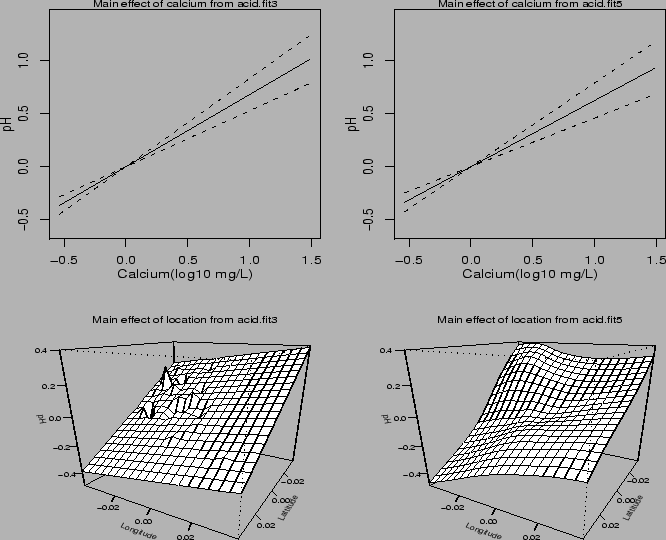Next: Wisconsin Epidemiological Study of Up: Examples Previous: Chickenpox Epidemic
Yuedong Wang 2004-05-19